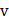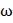# A disc of radiusrolls on a horizontal ground with linear accelerationand angular accelerationas shown in Figure. The magnitude of acceleration of pointasshown in the figure at an instant when its linear velocity isand angular velocity iswill bea)b)c)d)## Question ID - 100191 :- A disc of radiusrolls on a horizontal ground with linear accelerationand angular accelerationas shown in Figure. The magnitude of acceleration of pointasshown in the figure at an instant when its linear velocity isand angular velocity iswill bea)b)c)d)3537

(a)

FBD of pointIn the earth frame, we haveSo, resultant =Next Question :

A small object of uniform density rolls up a curved surface with an initial velocity. It reaches up to a maximum height ofw.r.t. the initial position. The object isa) Ring b) Solid sphere c) Hollow sphere d) disc